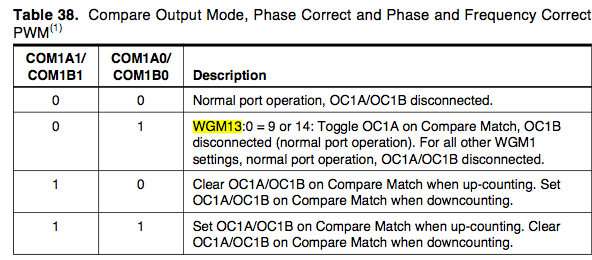###Author Topic: General Programming Help, how to set up pins?  (Read 1473 times)

0 Members and 1 Guest are viewing this topic.

####corrado33• Supreme Robot
•• Posts: 611##### General Programming Help, how to set up pins?
« on: April 02, 2010, 10:49:58 PM »
Hello again.  I know I have another thread right below this one, but I like to keep different topics separate for search-ability reasons.  So I'm going to try to write a program based on lots of timers and PWM stuff.

My first question is... how do you set certain pins for output/PWM/ anything.  I see this stuff all the time in sample programs, but I don't know exactly how to find it.

DDRF = 0b11111111;  //configure all F ports for output *
DDRK = 0b11111111;  //configure all K ports for output *
DDRB |= (1 << 0); // Set LED as output  **

*From AXON test code.
** From http://www.avrfreaks.net/index.php?name=PNphpBB2&file=viewtopic&t=50106&highlight=timers+tutorial

Question number 1:  The "DDRF" and "DDRK" come from the datasheet, correct?  How do you know to use those things?  Those are just bits right?

Question number 2:  This "0b11111111" is in...hex right?  What does it mean and why were the two things set to this number?

Question number 3:  Bits can only be "On" and "Off" right?  So when you are setting these things (which I'm assuming are bits) to these hex (I think) numbers, you're essentially just turning it on or off right?

Question number 4:  So to use certain features on a MCU, such as PWM or timers, you simply have to find what bits to turn on, and which to turn off, then your MCU will be set up as desired?  If this is true, how can you find which bits to turn on and off?  I'm assuming in the datasheet, but it's really hard to understand those things.

EDIT:  So, if I see something like this in my datasheet:Are the things on the left bits that I would set to get the desired operation on the right?

Question number 5:  Why in the first two examples is there an "=" but in the third one it's "|="  I know the "|=" means DDRB = DDRB | (1 << 0), but if both all three statements are essentially doing the same thing, why have two completely different ways of doing it?

Question number 6:  What's up with "(1 << 0)".  I know << means shift the bit one to the left... but there are no bits there, just two numbers...I thank anybody who can help me clear this stuff up...
« Last Edit: April 02, 2010, 11:01:28 PM by corrado33 »

####Pratheek

• Contest Winner
• Robot Overlord
•• Posts: 125##### Re: General Programming Help, how to set up pins?
« Reply #1 on: April 05, 2010, 10:09:31 AM »
Most of the answers to your questions can be found in datasheets and the numerous tutorials you will find on the internet.

Question number 1:  The "DDRF" and "DDRK" come from the datasheet, correct?  How do you know to use those things?  Those are just bits right?
Yes, they come from the datasheet and they are registers. The DDRx register is used to configure a particular port as input or output, where x is the port name.

Question number 2:  This "0b11111111" is in...hex right?  What does it mean and why were the two things set to this number?

Question number 3:  Bits can only be "On" and "Off" right?  So when you are setting these things (which I'm assuming are bits) to these hex (I think) numbers, you're essentially just turning it on or off right?
Actually, it is in binary. Anything that is followed by 0b is a binary number. The statement assigns the value of 11111111 = 1 (in decimal) to the DDRF register and makes the Port F as output.

Question number 4:  So to use certain features on a MCU, such as PWM or timers, you simply have to find what bits to turn on, and which to turn off, then your MCU will be set up as desired?  If this is true, how can you find which bits to turn on and off?  I'm assuming in the datasheet, but it's really hard to understand those things.

Yes, I agree. But you will get used to it.
Glance through the datasheet once, then read some online tutorial and then go through the datasheet throughly. The whole thing will become a whole lot easier and seem simpler.

Question number 5:  Why in the first two examples is there an "=" but in the third one it's "|="  I know the "|=" means DDRB = DDRB | (1 << 0), but if both all three statements are essentially doing the same thing, why have two completely different ways of doing it?

Question number 6:  What's up with "(1 << 0)".  I know << means shift the bit one to the left... but there are no bits there, just two numbers...

The | is the logical OR operator and the << operator is the left shift operator.
This tutorial explains these operators in detail - http://www.avrfreaks.net/index.php?name=PNphpBB2&file=viewtopic&t=37871